#Fourier Sine and Cosine SeriesRecall that the Fourier series of f(x) is defined by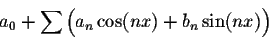where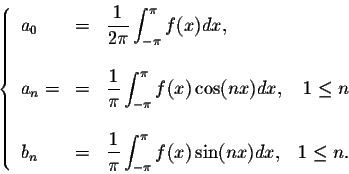We have the following result:

Theorem. Let f(x) be a function defined and integrable on interval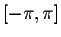.

(1)
If f(x) is even, then we have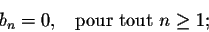and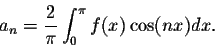(2)
If f(x) is odd, then we have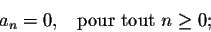and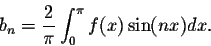This Theorem helps define the Fourier series for functions defined only on the interval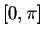. The main idea is to extend these functions to the intervaland then use the Fourier series definition.

Let f(x) be a function defined and integrable on. Set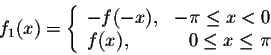and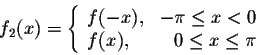Then f1 is odd and f2 is even. It is easy to check that these two functions are defined and integrable onand are equal to f(x) on. The function f1 is called the odd extension of f(x),
while f2 is called its even extension.

Definition. Let f(x), f1(x), and f2(x) be as defined above.

(1)
The Fourier series of f1(x) is called the Fourier Sine series of the function f(x), and is given by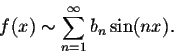where(2)
The Fourier series of f2(x) is called the Fourier Cosine series of the function f(x), and is given bywhere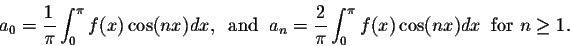Example. Find the Fourier Cosine series of f(x) = x for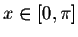.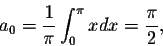and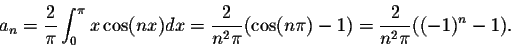Therefore, we have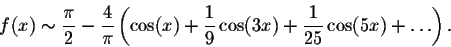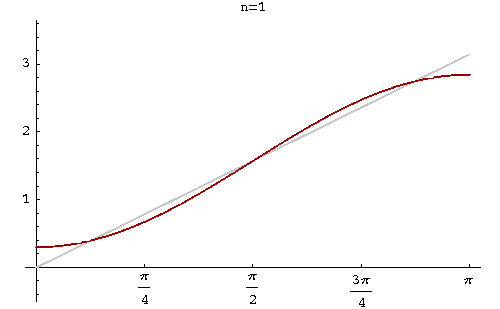Example. Find the Fourier Sine series of the function f(x) = 1 for.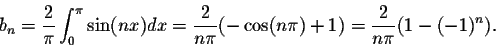Hence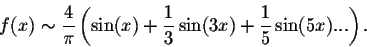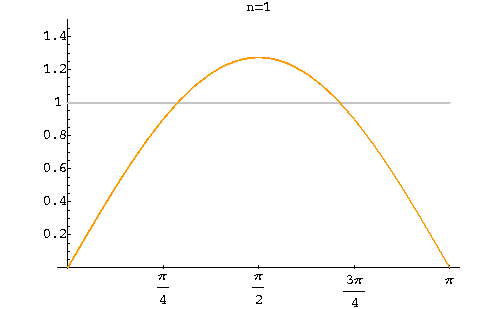Example. Find the Fourier Sine series of the function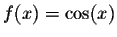for.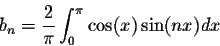which gives b1 = 0 and for n > 1, we obtain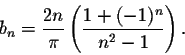Hence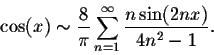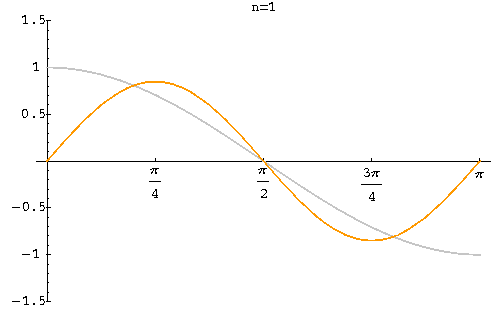Special Case of 2L-periodic functions.
As we did for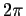-periodic functions, we can define the Fourier Sine and Cosine series for functions defined on the interval [-L,L]. First, recall the Fourier series of f(x)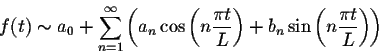where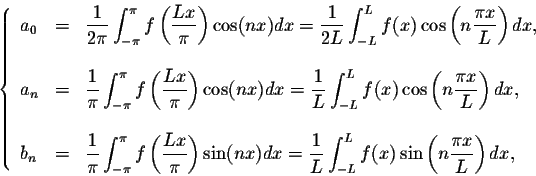for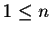.
1.
If f(x) is even, then bn = 0, for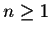. Moreover, we have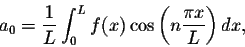and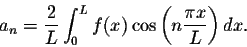Finally, we have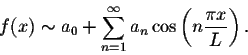2.
If f(x) is odd, then an = 0, for all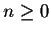, and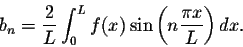Finally, we have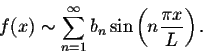The definitions of Fourier Sine and Cosine may be extended in a similar way.[Geometry] [Algebra] [Trigonometry ]
[Calculus] [Differential Equations] [Matrix Algebra]S.O.S MATH: Home Page

Do you need more help? Please post your question on our S.O.S. Mathematics CyberBoard.Author: M.A. Khamsi